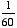index: click on a letter A B C D E F G H I J K L M N O P Q R S T U V W X Y Z A to Z index index: subject areas numbers & symbols sets, logic, proofs geometry algebra trigonometry advanced algebra & pre-calculus calculus advanced topics probability & statistics real world applications multimedia entrieswww.mathwords.com about mathwords website feedback

 Second A unit of angle measure equal toof a minute. There are 60 seconds in one minute and 3600 seconds in one degree. Seconds are indicated using the " symbol, so 12°45'33" means 12 degrees, 45 minutes, and 33 seconds, ordegrees.